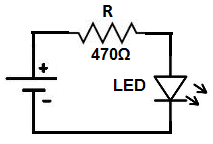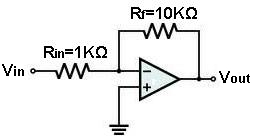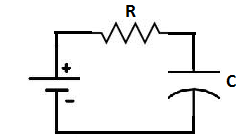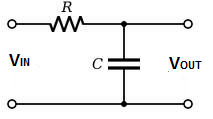﻿ What are Resistors Used For?

# What are Resistors Used For?Resistors, either standalone or used with other electronic components such as capacitors and inductors, have a wide variety of uses in circuits.

We will go over some of the most common ways resistors are used in circuits, shown below:

### 1) Resistors Lower Current In Circuits

One of the most important and widespread use of a resistor in a circuit is to lower current in a circuit. Chiefly and most importantly, resistors lower the amount of current passing through a circuit. Resistors decrease current by ohm's law, I=V/R. Therefore, the greater the resistance in a circuit, the lower the current. High currents can be very dangerous in circuits and can burn out and destroy electronic components. To counter this, resistors are placed in series with the sensitive electronic components to keep them from being destroyed by excess current.One such component where resistors almost always accompany are LEDs. LEDs are very sensitive components and a resistor placed in series them keeps them from being destroyed by excess power.

### 2) Resistors Are Used in Voltage Divider Circuits

Another use of resistors is in voltage divider circuits, which are circuits which divide voltage so that different parts of circuits receive different voltage values, as is needed, proportional to the value of resistances of the resistors in series. A voltage divider circuit divides voltage to various parts of a circuit based on the resistance values. Voltage is dropped across the resistors according to ohm's law, V=IR. So the greater the resistance value of a resistor, the greater the voltage which is dropped across it, while the lower the resistance, the less voltage is dropped across it.### 3)Resistors Are Used to Set the Gain of Op Amps

Resistors are used to set the gain of op amp circuits. If you can recall op amp circuits, a resistor, called a feedback resistor, is placed over the input and output of the op amp.This resistor along with the input resistor sets the gain of the op amp circuit, according to the formula, -RF/RIN, for inverting op amps.

### 4)Resistors Are Used in Timing Circuits

Resistors are used in timing circuits. When resistors and capacitors or resistors and inductors are placed in a circuit together, in series or in parallel, they form an RC or RL timing network.The greater the value of the resistance, the longer the time it takes for a capacitor or inductor to charge up. Conversely, the lower the value of the resistance, the shorter the time charging takes. This is because the greater the resistance, the more it slows down current. So resistors are heavily used in timing circuits.

### 5)Resistors Are Used in Filtering Circuits

Resistors are also used in all types of filtering circuits. Resistors, again, are used with the passive components, capacitors and inductors, to do this. They are used with these components to form low-pass and high-pass filters. Depending on the orientation or placement of the resistor in the circuit determines whether it acts as a high or low-pass filter. These serve as frequency filters in circuits; they allow a certain range of frequencies to pass through into the circuit and block all other frequency ranges. A low-pass filter is a filter which allows low frequencies to enter through. A high-pass filter is a filter which allows high frequencies to pass though, blocking low frequencies.### 6)Special Functions

Besides the basic types, many special types of resistors exist which have special functions. A varistor is a resistor which varies in values based on the voltage that drops across it. Thermistors are resistors which vary depending on the temperature they are exposed to. Potentiometers are resistors which can be varied to produce a range of different values which they are rated for. A photoresistor is a resistor which varies according to the amount of light that strikes its surface.

Related Resources